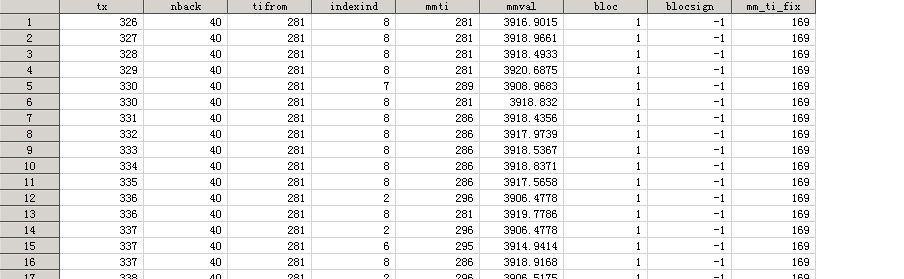## How to re-arrange column order ?!

How to re-assign column order in dataset?!

Proc Transpose generates column with order depends on the order of each value popping-up, see below.

Anyway to enforce order inside PROC?!1 ACCEPTED SOLUTION

Accepted Solutions

## Re: How to re-arrange column order ?!

You have many choices.

1) Is to add an extra TX group that has all of the names in the order you want as the first BY group so it will be used to define the variables in the order you want.  You can remove the extra observations with a WHERE= dataset option applied to the output dataset.  So if NAME is the variable you used to have PROC TRANSPOSE generate those names then perhaps something like this.

``````proc sql;
create table for_transpose as
select distinct min(tx),name
from have
order by 1,2
;
quit;
data for_transpose;
set for_transpose(in=in1) have;
by tx;
if in1 then call missing(tx);
run;
proc transpose data=for_transpose out=want(where=(not missing(tx))) ;
by tx;
var mmti;
id name;
run;``````

2) Add another step AFTER the transpose to set the variable order.

``````data want;
retain tx mmti_1-mmti_8;
set have;
run;``````

3) Don't use PROC TRANSPOSE at all.  Write your own data step and create your own variable names.

2 REPLIES 2

## Re: How to re-arrange column order ?!

Here is a similar quest solved, https://communities.sas.com/t5/SAS-Programming/Transpose-column-with-chronological-order/td-p/817427

But my source data does not guarantees that A[/1] shows up first for each by variable(s).## Re: How to re-arrange column order ?!

You have many choices.

1) Is to add an extra TX group that has all of the names in the order you want as the first BY group so it will be used to define the variables in the order you want.  You can remove the extra observations with a WHERE= dataset option applied to the output dataset.  So if NAME is the variable you used to have PROC TRANSPOSE generate those names then perhaps something like this.

``````proc sql;
create table for_transpose as
select distinct min(tx),name
from have
order by 1,2
;
quit;
data for_transpose;
set for_transpose(in=in1) have;
by tx;
if in1 then call missing(tx);
run;
proc transpose data=for_transpose out=want(where=(not missing(tx))) ;
by tx;
var mmti;
id name;
run;``````

2) Add another step AFTER the transpose to set the variable order.

``````data want;
retain tx mmti_1-mmti_8;
set have;
run;``````

3) Don't use PROC TRANSPOSE at all.  Write your own data step and create your own variable names.

Discussion stats
• 2 replies
• 209 views
• 0 likes
• 2 in conversation